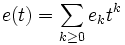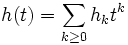# Elementary symmetric polynomial

Jump to: navigation, search

The homogeneous polynomials of this kind of any given degree form a basis for the space of all homogeneous symmetric polynomials of that degree

This construct is associated with, or parametrized by, an unordered integer partition

## Definition

The elementary symmetric polynomial in$m$ variables, associated with a partition$\lambda = (\lambda_1,\lambda_2,\ldots,\lambda_r)$ is defined as the product of the elementary symmetric polynomials associated with each$\lambda_i$. Here, the elementary symmetric polynomial associated with a positive integer$l$ is the sum of all monomials in the$m$ variables having degree$l$.

## Facts

### Nature of the polynomials

The elementary symmetric polynomials are all symmetric polynomials. In fact, the elementary symmetric polynomials corresponding to all tableaux with$n$ cells, in$m$ variables, form a basis for the space of all homogeneous symmetric polynomials in$m$ variables, of degree$n$.

### Generating function for the polynomials

PLACEHOLDER FOR INFORMATION TO BE FILLED IN: [SHOW MORE]

## Relation with other polynomials

### Complete symmetric polynomials

The map that changes basis from elementary symmetric polynomials to complete symmetric polynomials is in fact an involutive ring homomorphism. This follows from the fact that for any$t$, the product of the formal power series$e(t)$ and$h(-t)$ is 1, where:$e(t) = \sum_{k \ge 0} e_kt^k$

and$h(t) = \sum_{k \ge 0} h_kt^k$

### Schur polynomials

The matrix for changing basis from Elementary symmetric polynomials to Schur polynomials is the matrix of: Kostka numbers for the conjugate partition